Mathematics: CUET Mock Test - 2

# Mathematics: CUET Mock Test - 2

Test Description

## 40 Questions MCQ Test CUET Science Subjects Mock Tests | Mathematics: CUET Mock Test - 2

Mathematics: CUET Mock Test - 2 for Class 12 2023 is part of CUET Science Subjects Mock Tests preparation. The Mathematics: CUET Mock Test - 2 questions and answers have been prepared according to the Class 12 exam syllabus.The Mathematics: CUET Mock Test - 2 MCQs are made for Class 12 2023 Exam. Find important definitions, questions, notes, meanings, examples, exercises, MCQs and online tests for Mathematics: CUET Mock Test - 2 below.
Solutions of Mathematics: CUET Mock Test - 2 questions in English are available as part of our CUET Science Subjects Mock Tests for Class 12 & Mathematics: CUET Mock Test - 2 solutions in Hindi for CUET Science Subjects Mock Tests course. Download more important topics, notes, lectures and mock test series for Class 12 Exam by signing up for free. Attempt Mathematics: CUET Mock Test - 2 | 40 questions in 45 minutes | Mock test for Class 12 preparation | Free important questions MCQ to study CUET Science Subjects Mock Tests for Class 12 Exam | Download free PDF with solutions
 1 Crore+ students have signed up on EduRev. Have you?
Mathematics: CUET Mock Test - 2 - Question 1

### A particle moves in a straight-line OA; the distance of the particle from O at time t seconds is x ft, where x = a + bt + ct2 (a, b > 0). What will be the nature of motion of the particle when c = 0?

Detailed Solution for Mathematics: CUET Mock Test - 2 - Question 1

We have, x = a + bt + ct2  ……….(1)
Let, v and f be the velocity and acceleration of a particle at time t seconds.
Then, v = dx/dt = d(a + bt + ct2)/dt = b + ct   ……….(2)
And f = dv/dt = d(b + ct)/dt = c  ……….(3)
Clearly, when c = 0, then f = 0 that is, acceleration of the particle is zero.
Hence in this case the particle moves with an uniform velocity.

Mathematics: CUET Mock Test - 2 - Question 2

### A particle moving in a straight line traverses a distance x in time t. If t = x2/2 + x, then which one is correct?

Detailed Solution for Mathematics: CUET Mock Test - 2 - Question 2

We have, t = x2/2 + x
Therefore, dt/dx = 2x/2 + 1 = x + 1
Thus, if v be the velocity of the particle at time t, then
v = dx/dt = 1/(dt/dx)
= 1/(x + 1) = (x + 1)-1
Thus dv/dt = d((x + 1)-1)/dt
= (-1)(x + 1)-2 d(x + 1)/dt
= -1/(x + 1)2 * dx/dt
As, 1/(x + 1) = dx/dt,
So, -(dx/dt)2(dx/dt)
Or dv/dt = -v2*v  [as, dx/dt = v]
= -v3
We know, dv/dt = acceleration of a particle.
As, dv/dt is negative, so there is a retardation of the particle.
Thus, the retardation of the particle = -dv/dt = v3 = cube of the particle.

Mathematics: CUET Mock Test - 2 - Question 3

### A particle moves with uniform acceleration along a straight line and describes distances 21m, 43m and 91m at times 2, 4 and 7 seconds, respectively.What is the velocity of the particle in 3 seconds?

Detailed Solution for Mathematics: CUET Mock Test - 2 - Question 3

We assume that the particle moves with uniform acceleration 2f m/sec.
Let, x m be the distance of the particle from a fixed point on the straight line at time t seconds.
Let, v be the velocity of the particle at time t seconds, then,
So, dv/dt = 2f
Or ∫dv = ∫2f dt
Or v = 2ft + b   ……….(1)
Or dx/dt = 2ft + b
Or ∫dx = 2f∫tdt + ∫b dt
Or x = ft2 + bt + a   ……….(2)
Where, a and b are constants of integration.
Given, x = 21, when t = 2; x = 43, when t = 4 and x = 91, when t = 7.
Putting these values in (2) we get,
4f + 2b + a = 21   ……….(3)
16f + 4b + a = 43   ……….(4)
49f + 7b + a = 91  ……….(5)
Solving (3), (4) and (5) we get,
a = 7, b = 5 and f = 1
Therefore, from (2) we get,
x = t2 + 5t + 7
Putting t = 3, f = 1 and b = 5 in (1),
We get, the velocity of the particle in 3 seconds,
= [v]t = 3 = (2*1*3 + 5)m/sec = 11m/sec.

Mathematics: CUET Mock Test - 2 - Question 4

The distance x of a particle moving along a straight line from a fixed point on the line at time t after start is given by t = ax2 + bx + c (a, b, c are positive constants). If v be the velocity of the particle and u(≠0) be the initial velocity of the particle then which one is correct?

Detailed Solution for Mathematics: CUET Mock Test - 2 - Question 4

We have, t = ax2 + bx + c  ……….(1)
Differentiating both sides of (1) with respect to x we get,
dt/dx = d(ax2 + bx + c)/dx = 2ax + b
Thus, v = velocity of the particle at time t
= dx/dt = 1/(dt/dx) = 1/(2ax + b) = (2ax + b)-1  ……….(2)
Initially, when t = 0 and v = u, let x = x0; hence, from (1) we get,
ax02 + bx0 + c = 0
Or ax02 + bx0 = -c   ……….(3)
And from (2) we get, u = 1/(2ax0 + b)
Thus, 1/v2 – 1/u2 = (2ax + b)2 – (2ax0 + b)2
= 4a2x2 + 4abx – 4a2x02 – 4abx0
= 4a2x2 + 4abx – 4a(ax02 – bx0)
= 4a2x2 + 4abx – 4a(-c)   [using (3)]
= 4a(ax2 + bx + c)
Or 1/v2 – 1/u2 = 4at

Mathematics: CUET Mock Test - 2 - Question 5

A particle moves in a straight line and its velocity v at time t seconds is given byv = 3t2 – 4t + 5 cm/second. What will be the distance travelled by the particle during first 3 seconds after the start?

Detailed Solution for Mathematics: CUET Mock Test - 2 - Question 5

Let, x be the distance travelled by the particle in time t seconds.
Then, v = dx/dt = 3t2 – 4t + 5
Or ∫dx = ∫ (3t2 – 4t + 5)dt
So, on integrating the above equation, we get,
x = t3 – 2t2 + 5t + c where, c is a constant.  ……….(1)
Therefore, the distance travelled by the particle at the end of 3 seconds,
= [x]t = 3 – [x]t = 0
= (33 – 2*32 + 5*3 + c) – c [using (1)]
= 24 cm.

Mathematics: CUET Mock Test - 2 - Question 6

A particle moves with uniform acceleration along a straight line and describes distances 21m, 43m and 91m at times 2, 4 and 7 seconds,respectively.What is the distance described by the particle in 3 seconds?

Detailed Solution for Mathematics: CUET Mock Test - 2 - Question 6

We assume that the particle moves with uniform acceleration 2f m/sec.
Let, x m be the distance of the particle from a fixed point on the straight line at time t seconds.
Let, v be the velocity of the particle at time t seconds, then,
So, dv/dt = 2f
Or ∫dv = ∫2f dt
Or v = 2ft + b  ……….(1)
Or dx/dt = 2ft + b
Or ∫dx = 2f∫tdt + ∫b dt
Or x = ft2 + bt + a   ……….(2)
Where, a and b are constants of integration.
Given, x = 21, when t = 2; x = 43, when t = 4 and x = 91, when t = 7.
Putting these values in (2) we get,
4f + 2b + a = 21  ……….(3)
16f + 4b + a = 43  ……….(4)
49f + 7b + a = 91   ……….(5)
Solving (3), (4) and (5) we get,
a = 7, b = 5 and f = 1
Therefore, from (2) we get,
x = t2 + 5t + 7
Therefore, the distance described by the particle in 3 seconds,
= [x]t = 3 = (32 + 5*3 + 7)m = 31m

Mathematics: CUET Mock Test - 2 - Question 7

A particle moves in a horizontal straight line under retardation kv3, where v is the velocity at time t and k is a positive constant. If initial velocity be u and x be the displacement at time,then which one is correct?

Detailed Solution for Mathematics: CUET Mock Test - 2 - Question 7

Since the particle is moving in a straight line under a retardation kv3, hence, we have,
dv/dt = -kv3   ……….(1)
Or dv/v3 = -k dt
Or ∫v-3 dv = -k∫dt
Or v-3+1/(-3 + 1) = -kt – c [c = constant of integration]
Or 1/2v2 = kt + c   ……….(2)
Given, u = v when, t = 0; hence, from (2) we get,
1/2u2 = c
Thus, putting c = 1/2u2 in (2) we get,
1/2v2 = kt + 1/2u2
Or 1/v2 = 1/u2 + 2kt

Mathematics: CUET Mock Test - 2 - Question 8

The distance s of a particle moving along a straight line from a fixed-pointO on the line at time t seconds after start is given by x = (t – 1)2(t – 2)2. What will be the distance of the particle from O when its velocity is zero?

Detailed Solution for Mathematics: CUET Mock Test - 2 - Question 8

Let v be the velocity of the particle at time t seconds after start (that is at a distance s from O). Then,
v = ds/dt = d[(t – 1)2(t – 2)2]/dt
Or v = (t – 2)(3t – 4)
Clearly, v = 0, when (t – 2)(3t – 4) = 0
That is, when t = 2
Or 3t – 4 = 0 i.e., t = 4/3
Now, s = (t – 1)(t – 2)2
Therefore, when t = 4/3, then s = (4/3 – 1)(4/3 – 2)2 = 4/27
And when t = 2, then s =(2 – 1)(2 – 2)2 = 0
Therefore, the velocity of the particle is zero, when its distance from O is 4/27 units and when it is at O.

Mathematics: CUET Mock Test - 2 - Question 9

What will be the nature of the equation sin(x + α)/sin(x + β)?

Detailed Solution for Mathematics: CUET Mock Test - 2 - Question 9

Let, y = sin(x + α)/sin(x + β)
Then,
dy/dx = [cos(x + α)sin(x + β) – sin(x + α)cos(x + β)]/sin2(x + β)
= sin(x+β – x-α)/sin2(x + β)
Or sin(β – α)/sin2(x + β)
So, for minimum or maximum value of x we have,
dy/dx = 0
Or sin(β – α)/sin2(x + β) = 0
Or sin(β – α) = 0 ……….(1)
Clearly, equation (1) is independent of x; hence, we cannot have a real value of x as root of equation (1).
Therefore, y has neither a maximum or minimum value.

Mathematics: CUET Mock Test - 2 - Question 10

Given, f(x) = x3 – 12x2 + 45x + 8. What is the maximum value of f(x)?

Detailed Solution for Mathematics: CUET Mock Test - 2 - Question 10

We have, f(x) = x3 – 12x2 + 45x + 8 ……….(1)
Differentiating both sides of (1) with respect to x we
f’(x) = 3x2 – 24x + 45
3x2 – 24x + 45 = 0
Or x2 – 8x + 15 = 0
Or (x – 3)(x – 5) = 0
Thus, either x – 3 = 0 i.e., x = 3 or x – 5 = 0 i.e., x = 5
Therefore, f’(x) = 0 for x = 3 and x = 5.
If h be a positive quantity, however small, then,
f’(3 – h) = 3*(3 – h – 3)(3 – h – 5) = 3h(h + 2) = positive.
f’(3 + h) = 3*(3 + h – 3)(3 + h – 5) = 3h(h – 2) = negative.
Clearly, f’(x) changes sign from positive on the left to negative on the right of the point x = 3.
So, f(x) has maximum at 3.
Putting, x = 3 in (1)
Thus, its maximum value is,
f(3) = 33 – 12*32 + 45*3 + 8 = 62.

Mathematics: CUET Mock Test - 2 - Question 11

Given, f(x) = x3 – 12x2 + 45x + 8. What is the minimum value of f(x)?

Detailed Solution for Mathematics: CUET Mock Test - 2 - Question 11

We have, f(x) = x3 – 12x2 + 45x + 8 ……….(1)
Differentiating both sides of (1) with respect to x we
f’(x) = 3x2 – 24x + 45
3x2 – 24x + 45 = 0
Or x2 – 8x + 15 = 0
Or(x – 3)(x – 5) = 0
Thus, either x – 3 = 0 i.e., x = 3 or x – 5 = 0 i.e., x = 5
Therefore, f’(x) = 0 for x = 3 and x = 5.
If h be a positive quantity, however small, then,
f’(5 – h) = 3*(5 – h – 3)(5 – h – 5) = -3h(2 – h) = negative.
f’(5 + h) = 3*(5 + h – 3)(5 + h – 5) = 3h(2 + h) = positive.
Clearly, f’(x) changes sign from negative on the left to positive on the right of the point x = 5.
So, f(x) has minimum at 5.
Putting, x = 5 in (1)
Thus, its maximum value is,
f(3) = 53 – 12*52 + 45*5 + 8 = 58.

Mathematics: CUET Mock Test - 2 - Question 12

A particle is moving in a straight line and its distance x from a fixed point on the line at any time t seconds is given by, x = t4/12 – 2t3/3 + 3t2/2 + t + 15. At what time is the velocity minimum?

Detailed Solution for Mathematics: CUET Mock Test - 2 - Question 12

Assume that the velocity of the particle at time t second is vcm/sec.
Then, v = dx/dt = 4t3/12 – 6t2/3 + 6t/2 + 1
So, v = dx/dt = t3/3 – 2t2/ + 3t + 1
Thus, dv/dt = t2 – 4t + 3
And d2v/dt2 = 2t – 4
For maximum and minimum value of v we have,
dv/dt = 0
Or t2 – 4t + 3 = 0
Or (t – 1)(t – 3) = 0
Thus, t – 1 = 0 i.e., t = 1 Or t – 3 = 0 i.e., t = 3
Now, [d2v/dt2]t = 3 = 2*3 – 4 = 2 > 0
Thus, v is minimum at t = 3.

Mathematics: CUET Mock Test - 2 - Question 13

Given, f(x) = x3 – 12x2 + 45x + 8. At which point does f(x) has its maximum?

Detailed Solution for Mathematics: CUET Mock Test - 2 - Question 13

We have, f(x) = x3 – 12x2 + 45x + 8 ……….(1)
Differentiating both sides of (1) with respect to x we
f’(x) = 3x2 – 24x + 45
3x2 – 24x + 45 = 0
Or x2 – 8x + 15 = 0
Or (x – 3)(x – 5) = 0
Thus, either x – 3 = 0 i.e., x = 3 or x – 5 = 0 i.e., x = 5
Therefore, f’(x) = 0 for x = 3 and x = 5.
If h be a positive quantity, however small, then,
f’(3 – h) = 3*(3 – h – 3)(3 – h – 5) = 3h(h + 2) = positive.
f’(3 + h) = 3*(3 + h – 3)(3 + h – 5) = 3h(h – 2) = negative.
Clearly, f’(x) changes sign from positive on the left to negative on the right of the point x = 3.
So, f(x) has maximum at 3.

Mathematics: CUET Mock Test - 2 - Question 14

Given, f(x) = x3 – 12x2 + 45x + 8. At which point does f(x) has its minimum?

Detailed Solution for Mathematics: CUET Mock Test - 2 - Question 14

We have, f(x) = x3 – 12x2 + 45x + 8 ……….(1)
Differentiating both sides of (1) with respect to x we
f’(x) = 3x2 – 24x + 45
3x2 – 24x + 45 = 0
Or x2 – 8x + 15 = 0
Or (x – 3)(x – 5) = 0
Thus, either x – 3 = 0 i.e., x = 3 or x – 5 = 0 i.e., x = 5
Therefore, f’(x) = 0 for x = 3 and x = 5.
If h be a positive quantity, however small, then,
f’(5 – h) = 3*(5 – h – 3)(5 – h – 5) = -3h(2 – h) = negative.
f’(5 + h) = 3*(5 + h – 3)(5 + h – 5) = 3h(2 + h) = positive.
Clearly, f’(x) changes sign from negative on the left to positive on the right of the point x = 5.
So, f(x) has minimum at 5.

Mathematics: CUET Mock Test - 2 - Question 15

At which point does f(x) = |x – 1| has itslocal minimum?

Detailed Solution for Mathematics: CUET Mock Test - 2 - Question 15

The given function is f(x) = ∣x − 1∣, x ∈ R.
It is known that a function f is differentiable at point x = c in its domain if both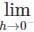− hf(c + h) – f(c)
And
l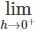+ hf(c + h) – f(c) are finite and equal.
To check the differentiability of the function at x = 1,
LHS,
Consider the left hand limit of f at x=1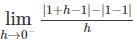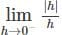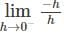= −1
RHS,
Consider the right hand limit of f at x − 1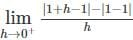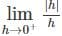= 1
Since the left and right hand limits of f at x = 1 are not equal, f is not differentiable at x = 1.
As, LHS = -1 and RHS = 1, it is clear that, f’(1) < 0 on the left of x = 1 and f’(x) > 0 on the right of the point x = 1.
Hence, f’(x) changes sign, from negative on the left to positive on the right of the point x = 1.
Therefore, f(x) has a local minima at x = 1.

Mathematics: CUET Mock Test - 2 - Question 16

A particle is moving in a straight line and its distance x from a fixed point on the line at any time t seconds is given by, x = t4/12 – 2t3/3 + 3t2/2 + t + 15. What is the minimum velocity?

Detailed Solution for Mathematics: CUET Mock Test - 2 - Question 16

Assume that the velocity of the particle at time t second is vcm/sec.
Then, v = dx/dt = 4t3/12 – 6t2/3 + 6t/2 + 1
So, v = dx/dt = t3/3 – 2t2/ + 3t + 1
Thus, dv/dt = t2 – 4t + 3
And d2v/dt2 = 2t – 4
For maximum and minimum value of v we have,
dv/dt = 0
Or t2 – 4t + 3 = 0
Or (t – 1)(t – 3) = 0
Thus, t – 1 = 0 i.e., t = 1 Or t – 3 = 0 i.e., t = 3
Now, [d2v/dt2]t = 3 = 2*3 – 4 = 2 > 0
Thus, v is minimum at t = 3.
Putting t = 3 in (1) we get,
33/3 – 2(3)2/ + 3(3) + 1
= 1 cm/sec.

Mathematics: CUET Mock Test - 2 - Question 17

What will be the equation of the normal to the parabola y2 = 5x that makes an angle 45° with the x axis?

Detailed Solution for Mathematics: CUET Mock Test - 2 - Question 17

The equation of the given parabola is, y2 = 5x ……….(1)
Differentiating both sides of (1) with respect to y, we get,
2y = 5(dx/dy)
Or dx/dy = 2y/5
Take any point P((5/4)t2, (5/2)t). Then, the normal to the curve (1) at P is,
-[dx/dy]P = -(2*5t/2)/5 = -t
By the question, slope of the normal to the curve (1) at P is tan45°.
Thus, -t = 1
Or t = -1
So, the required equation of normal is,
y – 5t/2 = -t(x – 5t2/4)
Simplifying further we get,
4(x – y) = 15

Mathematics: CUET Mock Test - 2 - Question 18

What will be the equation of the normal to the parabola y2 = 3x which is perpendicular to the line y = 2x + 4?

Detailed Solution for Mathematics: CUET Mock Test - 2 - Question 18

Given, y2 = 3x ……….(1) and y = 2x + 4 ……….(2)
Differentiating both sides of (1) with respect to y we get,
2y = 3(dx/dy)
Or dx/dy = 2y/3
Let P (x1, y1) be any point on the parabola (1). Then the slope of the normal to the parabola (1) at point P is
-[dx/dy]P = -2y1/3
If the normal at the point P to the parabola (1) be perpendicular to the line (2) then we must have,
-2y1/3*2 = -1
Since the slope of the line (2) is 2
Or y1 = 3/4
Since the point P(x1, y1) lies on (1) hence,
y12 = 3x1
As, y1 = 3/4, so, x1 = 3/16
Therefore, the required equation of the normal is
y – y1 = -(2y1)/3*(x – x1)
Putting the value of x1 and y1 in the above equation we get,
16x + 32y = 27.

Mathematics: CUET Mock Test - 2 - Question 19

What will be the length of a tangent from the point (7, 2) to the circle 2x2 + 2y2 + 5 x + y = 15?

Detailed Solution for Mathematics: CUET Mock Test - 2 - Question 19

The given equation of the circle is,
2x2 + 2y2 + 5 x + y – 15 = 0
Or x2 + y2 + 5/2 x + y/2 – 15/2 = 0 ………..(1)
The required length of the tangent drawn from the point (7, 2) to the circle (1) is,
√(72 + 22 + 5/2 (7) + 1/2 – 15/2)
= √(49 + 4 + 35/2 + 1 – 15/2)
= √64
= 8 units.

Mathematics: CUET Mock Test - 2 - Question 20

What will be the equation of the circle which touches the line x + 2y + 5 = 0 and passes through the point of intersection of the circle x2 + y2 = 1 and x2 + y2 + 2x + 4y + 1 = 0?

Detailed Solution for Mathematics: CUET Mock Test - 2 - Question 20

The equation of any circle through the points of intersection of the given circle is,
x2 + y2 + 2x + 4y + 1 + k(x2 + y2 – 1) = 0
x2 + y2 + 2x(1/(k + 1)) + 2*2y/(k + 1) + (1 – k)/(1 + k) = 0
Clearly, the co-ordinates of the center of the circle (1) are, (-1/(1 + k), -2/(1 + k)) and its radius,
= √[(1/(1 + k))2 + (2/(1 + k))2 – ((1 – k)]/(1 + k))
= √(4 + k2)/(1 + k)
Clearly, the line x + 2y + 5 = 0 is tangent to the circle (1), hence, the perpendicular distance of the line from the center of the circle = radius of the circle
± (-1/(1 + k))– 2(2/(1 + k)) + 5/ √(12 + 22) = √(4 + k2)/(1 + k)
Or ±(5k/√5) = √(4 + k2)
Or 5k2 = 4 + k2
Or 4k2 = 4
Or k = 1 [as, k ≠ -1]
Putting k = 1 in (1), equation of the given circle is,
x2 + y2 + x + 2y = 0

Mathematics: CUET Mock Test - 2 - Question 21

If the curves x2/a + y2/b = 1 and x2/c + y2/d = 1 intersect at right angles, then which one is the correct relation?

Detailed Solution for Mathematics: CUET Mock Test - 2 - Question 21

We have, x2/a + y2/b = 1 ……….(1)
and
x2/c + y2/d = 1 ……….(2)
Let, us assume curves (1) and (2) intersect at (x1, y1). Then
x12/a + y12/b = 1 ……….(3)
and
x12/c + y12/d = 1 ……….(4)
Differentiating both side of (1) and (2) with respect to x we get,
2x/a + 2y/b(dy/dx) = 0
Or dy/dx = -xb/ya
Let, m1 and m2 be the slopes of the tangents to the curves (1) and (2) respectively at the point (x1, y1); then,
m1 = [dy/dx](x1, y1) = -(bx1/ay1) and m2 = [dy/dx](x1, y1) = -(dx1/cy1)
By question as the curves (1) and (2) intersects at right angle, so, m1m2 = -1
Or -(bx1/ay1)*-(dx1/cy1) = -1
Or bdx12 = -acy12 ……….(5)
Now, (3) – (4) gives,
bdx12(c – a) = acy12(d – b) ……….(6)
Dividing (6) by (5) we get,
c – a = d – b
Or a – b = c – d.

Mathematics: CUET Mock Test - 2 - Question 22

What will be the co-ordinates of the foot of the normal to the parabola y2 = 5x that makes an angle 45° with the x axis?

Detailed Solution for Mathematics: CUET Mock Test - 2 - Question 22

The equation of the given parabola is, y2 = 5x ……….(1)
Differentiating both sides of (1) with respect to y, we get,
2y = 5(dx/dy)
Or dx/dy = 2y/5
Take any point P((5/4)t2, (5/2)t). Then, the normal to the curve (1) at P is,
-[dx/dy]P = -(2*5t/2)/5 = -t
By the question, slope of the normal to the curve (1) at P is tan45°.
Thus, -t = 1
Or t = -1
So, the required equation of normal is,
y – 5t/2 = -t(x – 5t2/4)
Simplifying further we get,
4(x – y) = 15
The co-ordinates of the foot of the normal are, P((5/4)t2, (5/2)t).
As t = 1, so putting the value of t = 1, we get,
P = (5/4, 5/2).

Mathematics: CUET Mock Test - 2 - Question 23

What will be the co-ordinates of the foot of the normal to the parabola y2 = 3x which is perpendicular to the line y = 2x + 4?

Detailed Solution for Mathematics: CUET Mock Test - 2 - Question 23

Given, y2 = 3x ……….(1) and y = 2x + 4 ……….(2)
Differentiating both sides of (1) with respect to y we get,
2y = 3(dx/dy)
Or dx/dy = 2y/3
Let P (x1, y1) be any point on the parabola (1). Then the slope of the normal to the parabola (1) at point P is
-[dx/dy]P = -2y1/3
If the normal at the point P to the parabola (1) be perpendicular to the line (2) then we must have,
-2y1/3*2 = -1
Since the slope of the line (2) is 2
Or y1 = 3/4
Since the point P(x1, y1) lies on (1) hence,
y12 = 3x1
As, y1 = 3/4, so, x1 = 3/16
Therefore, the required equation of the normal is
y – y1 = -(2y1)/3*(x – x1)
Putting the value of x1 and y1 in the above equation we get,
16x + 32y = 27
And the coordinates of the foot of the normal are (x1, y1) = (3/16, 3/4)

Mathematics: CUET Mock Test - 2 - Question 24

If the normal to the ellipse x2 + 3y2 = 12 at the point be inclined at 60° to the major axis, then at what angle does the line joining the curve to the point is inclined to the same axis?

Detailed Solution for Mathematics: CUET Mock Test - 2 - Question 24

Given, x2 + 3y2 = 12 Or x2/12 + y2/4 = 1
Differentiating both sides of (1) with respect to y we get,
2x*(dx/dy) + 3*2y = 0
Or dx/dy = -3y/x
Suppose the normal to the ellipse (1) at the point P(√12cosθ, 2sinθ) makes an angle 60° with the major axis. Then, the slope of the normal at P is tan60°
Or -[dx/dy]P = tan60°
Or -(-(3*2sinθ)/√12cosθ) = √3
Or √3tanθ = √3
Or tanθ = 1
Now the centre of the ellipse (1) is C(0, 0)
Therefore, the slope of the line CP is,
(2sinθ – 0)/(√12cosθ – 0) = (1/√3)tanθ = 1/√3 [as, tanθ = 1]
Therefore, the line CP is inclined at 30° to the major axis.

Mathematics: CUET Mock Test - 2 - Question 25

What will be the value of angle between the curves x2 - y2 = 2a2 and xv + y2 = 4a2?

Detailed Solution for Mathematics: CUET Mock Test - 2 - Question 25

x2 – y2 = 2a2 ……….(1) and x2 + y2 = 4a2 ……….(2)
Adding (1) and (2) we get, 2x2 = 6a2
Again, (2) – (1) gives,
2y2 = 2a2
Therefore, 2x2 * 2y2 = 6a2 * 2a2
4x2y2 = 12a2
Or x2y2 = 3a4
Or 2xy = ±2√3
Differentiating both side of (1) and (2) with respect to x we get,
2x – 2y(dy/dx) = 0
Or dy/dx = x/y
And 2x + 2y(dy/dx) = 0
Ordy/dx = -x/y
Let (x, y) be the point of intersection of the curves(1) and (2) and m1 and m2 be the slopes of the tangents to the curves (1) and (2) respectively at the point (x, y); then,
m1 = x/y and m2 = -x/y
Now the angle between the curves (1) and (2) means the angle between the tangents to the curve at their point of intersection.
Therefore, if θ is the required angle between the curves (1) and (2), then
tanθ = |(m1 – m2)/(1 + m1m2)|
Putting the value of m1, m2 in the above equation we get,
tanθ = |2xy/(y2 – x2)|
As, 2xy = ±2√3a2 and x2 – y2 = 2a2
tanθ = |±2√3a2/-2a2|
Or tanθ = √3
Thus, θ = π/3.

Mathematics: CUET Mock Test - 2 - Question 26

What is the other name for Bernoulli trials?

Detailed Solution for Mathematics: CUET Mock Test - 2 - Question 26

Bernoulli trials is also called a Dichotomous experiment and is repeated n times. If in each trial the probability of success is constant, then such trials are called Bernoulli trails.

Mathematics: CUET Mock Test - 2 - Question 27

(a1, a2) ∈R implies that (a2, a1) ∈ R, for all a1, a2∈A. This condition is for which of the following relations?

Detailed Solution for Mathematics: CUET Mock Test - 2 - Question 27

The above is a condition for a symmetric relation.
For example, a relation R on set A = {1,2,3,4} is given by R={(a,b):a+b=3, a>0, b>0}
1+2 = 3, 1>0 and 2>0 which implies (1,2) ∈ R.
Similarly, 2+1 = 3, 2>0 and 1>0 which implies (2,1)∈R. Therefore both (1, 2) and (2, 1) are converse of each other and is a part of the relation. Hence, they are symmetric.

Mathematics: CUET Mock Test - 2 - Question 28

Bernoulli trials are also called as _____ or _____ questions.

Detailed Solution for Mathematics: CUET Mock Test - 2 - Question 28

Bernoulli trials is also called a Dichotomous experiment and is repeated n times. Bernoulli trials is also called as a ‘yes’ or ‘no’ questions because it has only two outcomes, those are ‘success’ and ‘failure’.

Mathematics: CUET Mock Test - 2 - Question 29

What is the value of sin-1(sin 6)?

Detailed Solution for Mathematics: CUET Mock Test - 2 - Question 29

We know that sin(x) = sin(2A * π + x) where A can be positive or negative integer.
If A is -1, then sin(6) = sin(-2π + 6);
If A is 1, then sin(6) = sin(2π + 6);

Mathematics: CUET Mock Test - 2 - Question 30

What is the formula for binomial distribution?

Detailed Solution for Mathematics: CUET Mock Test - 2 - Question 30

Formula for binomial distribution is P [X = x] = nCx px qn-x
Where n is the number of trials, x is the number of successes and (n-x) is failures.

Mathematics: CUET Mock Test - 2 - Question 31

P(x; μ) = (e) (μx) / x! is the formula for _____

Detailed Solution for Mathematics: CUET Mock Test - 2 - Question 31

Poisson distribution shows the number of times an event is likely to occur within a specified time. The Poisson distribution probability formula is P(x; μ) = (e) (μx) / x!

Mathematics: CUET Mock Test - 2 - Question 32

P [X = x] = nCx px qn-x is the formula for _____

Detailed Solution for Mathematics: CUET Mock Test - 2 - Question 32

Formula for binomial distribution is P [X = x] = nCx px qn-x
Where n is the number of trials, x is the number of successes and (n-x) is failures.

Mathematics: CUET Mock Test - 2 - Question 33

The term Bernoulli trials is termed after which swiss mathematician?

Detailed Solution for Mathematics: CUET Mock Test - 2 - Question 33

Bernoulli trials is termed after swiss mathematician Jacob Bernoulli. Bernoulli trials is also called a Dichotomous experiment and is repeated n times. If in each trial the probability of success is constant, then such trials are called Bernoulli trails.

Mathematics: CUET Mock Test - 2 - Question 34

How many outcomes can a Bernoulli trial have?

Detailed Solution for Mathematics: CUET Mock Test - 2 - Question 34

Bernoulli trial has only two possible outcomes and is mutually exclusive. Those two outcomes are ‘success’ and ‘failure’. So, it is also called as a ‘yes’ or ‘no’ question.

Mathematics: CUET Mock Test - 2 - Question 35

The Poisson distribution comes under which probability distribution?

Detailed Solution for Mathematics: CUET Mock Test - 2 - Question 35

Poisson distribution shows the number of times an event is likely to occur within a specified time. It is used only for independent events that occur at a constant rate within a given interval of time.

Mathematics: CUET Mock Test - 2 - Question 36

What is the formula for the Poisson distribution probability?

Detailed Solution for Mathematics: CUET Mock Test - 2 - Question 36

Poisson distribution shows the number of times an event is likely to occur within a specified time. The Poisson distribution probability formula is P(x; μ) = (e) (μx) / x!

Mathematics: CUET Mock Test - 2 - Question 37

What will be the value of x + y + z if cos-1 x + cos-1 y + cos-1 z = 3π?

Detailed Solution for Mathematics: CUET Mock Test - 2 - Question 37

The equation is cos-1 x + cos-1 y + cos-1 z = 3π
This means cos-1 x = π, cos-1 y = π and cos-1 z = π
This will be only possible when it is in maxima.
As, cos-1 x = π so, x = cos-1 π = -1 similarly, y = z = -1
Therefore, x + y + z = -1 -1 -1
So, x + y + z = -3.

Mathematics: CUET Mock Test - 2 - Question 38

Method in which the previously calculated probabilities are revised with values of new probability is called ____

Detailed Solution for Mathematics: CUET Mock Test - 2 - Question 38

Bayes theorem is the method in which the calculated probabilities are revised with values of new probabilities, whereas Updation theorem, Revision theorem and Dependent theorem are not related to the concept of probability.

Mathematics: CUET Mock Test - 2 - Question 39

Formula for conditional probability P(A | B) is _______

Detailed Solution for Mathematics: CUET Mock Test - 2 - Question 39

Conditional probability P(A | B) indicates the probability of event ‘A’ happening given that event B has happened.
Which in formula can be written as P(A|B) = P(A∩B)/P(B).
Whereas formula’s P(A | B) = P(A∩B)/P(A), P(A | B) = P(A)/P(B), P(A|B) = P(B)/P(A) doesn’t satisfies the specified conditions.

Mathematics: CUET Mock Test - 2 - Question 40

A man is known to speak truth 3 out of 4 times. He throws a die and reports that it is a six. Find the probability that it is actually a six.

Detailed Solution for Mathematics: CUET Mock Test - 2 - Question 40

Let E = event that the man reports that six in the throwing of the die and let, S1 = event that six occurs and S2 = event that six does not occur.
P(S1) = Probability that six occurs = 1/6.
P(S2) = Probability that six does not occur = 5/6.
Also, P(E|S1) = P(Probability that man reports six occurs when six actually has occurred on the die) = 3/4.
P(E|S2) = P(Probability that man reports six occurs when six not actually occurred on the die) =
1 – 3/4 = 1/4.
By using Bayes’ theorem,
P(S2 | E) = P(S1)P(E | S1)/(P(S1)P(E│S1)+P(S2)P(E | S2))
= (1/6 × 3/4) / ((1/6 × 3/4)) + (5/6 × 1/4)) = 3/8.

## CUET Science Subjects Mock Tests

60 tests
 Use Code STAYHOME200 and get INR 200 additional OFF Use Coupon Code
Information about Mathematics: CUET Mock Test - 2 Page
In this test you can find the Exam questions for Mathematics: CUET Mock Test - 2 solved & explained in the simplest way possible. Besides giving Questions and answers for Mathematics: CUET Mock Test - 2, EduRev gives you an ample number of Online tests for practice

60 tests